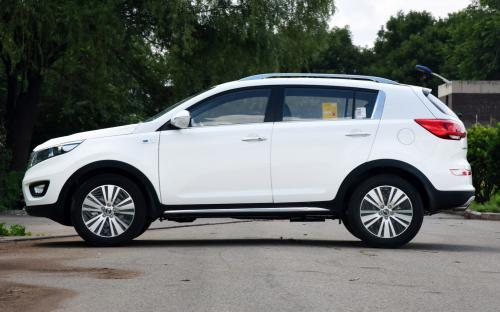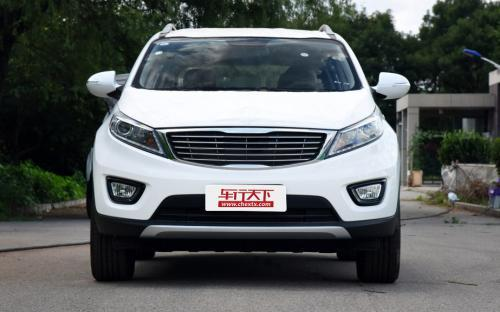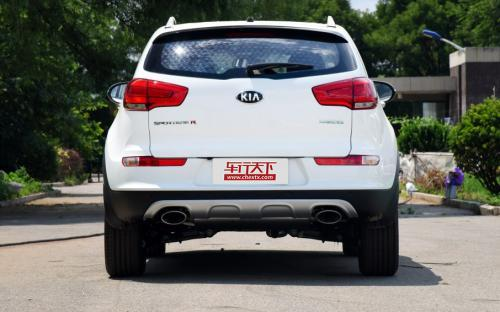### 东风悦达起亚 智跑0 种颜色可选2016款最低售价：14.48 万元起

4450(mm)1855(mm)1660(mm)##### 配置亮点：
• 胎压监测装置

• ISOFIX儿童座椅接口

• 车身稳定控制(ESC/ESP/DSC等)

• 电动天窗

• 定速巡航

• 后倒车雷达

• 真皮座椅

• GPS导航系统

• 氙气大灯

• 后视镜加热

• 提交
2016款 2.0L 自动两驱版DLX (115张)

东风悦达起亚 智跑 绕车实拍• 东风悦达起亚 智跑 在售车型

排量 车型 厂商指导价 本地最低报价 购车工具
2.0L
14.48万
2.0L 自动两驱版GL 6挡手自一体
15.88万
2.0L 自动两驱版GLS 6挡手自一体
17.28万
2.0L 自动两驱版DLX 6挡手自一体
17.78万
18.98万
2.4L
14.48万
2.0L 自动两驱版GL 6挡手自一体
15.88万
2.0L 自动两驱版GLS 6挡手自一体
17.28万
2.0L 自动两驱版DLX 6挡手自一体
17.78万
18.98万

东风悦达起亚 智跑 经销商

查看更多 >>

### 东风悦达起亚 智跑 动力加速

智跑 0-100公里加速时间分布在 0.0-秒 属于 超跑级

动力级别 加速时间 车型

东风悦达起亚 智跑 视频

东风悦达起亚 智跑 新闻资讯

# 试东风悦达起亚新款智跑 时尚和前卫并存

评测 超过7941次关注

从起亚智跑上市之初，无论是广告还是媒体的评测所给予其最多的形容词便是时尚和前卫，智跑一直以阳光大男孩般的形象出现在人们面前。当初厂商对于智跑目标客户群体...

# 起亚新款智跑实拍解析 重点变化在外观

导购 超过6075次关注

在2014年成都车展中，东风悦达起亚携新款智跑正式亮相。相比现款车型，新车采用了全新的前脸设计，整体造型更为个性，并且对于内饰，新款智跑也进行了一定调整。据...

# 心“试” 试驾东风悦达起亚2012款智跑

车系文章 超过2456次关注

“DESIGNKIA”是起亚打造精品之作的市场战略，智跑上市至今，在业界及市场都获得了非凡的成绩，2011年1-10月，东风悦达起亚累计销量已突破30万辆，成功跻身行业前十...

# 起亚2014款智跑价格公布 售16.48万元起

车系文章 超过2909次关注

今日，东风悦达起亚正式公布2014款智跑价格。新款智跑共将推出10款两个排量的车型，售价区间为16.48万-24.98万，其中大部分车型相比老款车型价格并没有变化，在车系...

# 日产新奇骏竞争力分析 东风悦达起亚智跑

车系文章 超过2205次关注

东风悦达起亚智跑与北京现代ix35基于同一平台打造，也是一款典型的城市SUV，其经典的虎啸式前脸设计已经深入人心。2014款智跑的上市在现有车型基础了对配置做了进一...

# 外观小幅修改 起亚新款智跑国内申报图

车系文章 超过3164次关注

近日，我们获得了一组疑似东风悦达起亚新款智跑的申报图，新车在外观方面进行了小幅修改，预计将于今年内正式上市。

# K4/新款智跑/小型SUV 起亚将推三款新车

车系文章 超过4009次关注

日前，我们通过对东风悦达起亚有限公司副总经理销售本部长林钟宪的专访中了解到，起亚在今年到明年将有3款新车型推出，包括K4、新款智跑以及全新小型SUV。

# 售16.48-24.98万 2014款智跑正式上市

车系文章 超过2451次关注

近日，东风起亚悦达正式公布了2014款起亚智跑的部分车型的售价，售价区间为16.48万-24.98万元。

猜你喜欢

﻿
• 快速找车
• 选择品牌
• 选择品牌
• A  奥迪
• A  阿斯顿·马丁
• A  阿尔法·罗密欧
• B  宝沃
• B  布加迪
• B  巴博斯
• B  保时捷
• B  宾利
• B  奔驰
• B  宝马
• B  本田
• B  别克
• B  标致
• B  比亚迪
• B  宝骏
• B  北汽制造
• B  北汽新能源
• B  北汽幻速
• B  北汽威旺
• B  北京汽车
• B  奔腾
• B  北汽绅宝
• C  长安
• C  长安商用
• C  长城
• C  昌河
• D  大众
• D  道奇
• D  DS
• D  东南
• D  东风风神
• D  东风风行
• D  东风小康
• D  东风风度
• D  东风
• F  福特
• F  丰田
• F  菲亚特
• F  法拉利
• F  福田
• F  福迪
• F  福汽启腾
• G  观致
• G  广汽传祺
• G  广汽吉奥
• G  GMC
• H  红旗
• H  汉腾汽车
• H  哈弗
• H  哈飞
• H  海格
• H  海马
• H  华颂
• H  黄海
• H  华泰
• H  恒天
• J  吉利汽车
• J  捷豹
• J  Jeep
• J  江淮
• J  江铃
• J  金杯
• J  九龙
• J  金旅
• K  凯翼
• K  凯迪拉克
• K  克莱斯勒
• K  科尼塞克
• K  卡威
• K  开瑞
• L  路虎
• L  林肯
• L  劳斯莱斯
• L  兰博基尼
• L  雷克萨斯
• L  铃木
• L  雷诺
• L  理念
• L  力帆
• L  莲花汽车
• L  猎豹
• L  路特斯
• L  陆风
• M  马自达
• M  MG
• M  MINI
• M  玛莎拉蒂
• M  摩根
• M  迈凯轮
• N  纳智捷
• O  欧宝
• O  讴歌
• O  欧朗
• Q  奇瑞
• Q  起亚
• Q  启辰
• R  日产
• R  荣威
• R  瑞麒
• S  三菱
• S  斯威汽车
• S  萨博
• S  smart
• S  斯柯达
• S  斯巴鲁
• S  思铭
• S  双龙
• S  上汽大通
• S  双环
• T  特斯拉
• T  腾势
• W  沃尔沃
• W  五菱汽车
• W  五十铃
• W  威兹曼
• W  威麟
• X  现代
• X  雪佛兰
• X  雪铁龙
• X  西雅特
• Y  一汽
• Y  英菲尼迪
• Y  英致
• Y  依维柯
• Y  野马汽车
• Y  永源
• Z  众泰
• Z  中华
• Z  中兴
• Z  知豆
• 选择车系
• 选择车系
• 车型对比
• 选择品牌
• 选择品牌
• A  奥迪
• A  阿斯顿·马丁
• A  阿尔法·罗密欧
• B  宝沃
• B  布加迪
• B  巴博斯
• B  保时捷
• B  宾利
• B  奔驰
• B  宝马
• B  本田
• B  别克
• B  标致
• B  比亚迪
• B  宝骏
• B  北汽制造
• B  北汽新能源
• B  北汽幻速
• B  北汽威旺
• B  北京汽车
• B  奔腾
• B  北汽绅宝
• C  长安
• C  长安商用
• C  长城
• C  昌河
• D  大众
• D  道奇
• D  DS
• D  东南
• D  东风风神
• D  东风风行
• D  东风小康
• D  东风风度
• D  东风
• F  福特
• F  丰田
• F  菲亚特
• F  法拉利
• F  福田
• F  福迪
• F  福汽启腾
• G  观致
• G  广汽传祺
• G  广汽吉奥
• G  GMC
• H  红旗
• H  汉腾汽车
• H  哈弗
• H  哈飞
• H  海格
• H  海马
• H  华颂
• H  黄海
• H  华泰
• H  恒天
• J  吉利汽车
• J  捷豹
• J  Jeep
• J  江淮
• J  江铃
• J  金杯
• J  九龙
• J  金旅
• K  凯翼
• K  凯迪拉克
• K  克莱斯勒
• K  科尼塞克
• K  卡威
• K  开瑞
• L  路虎
• L  林肯
• L  劳斯莱斯
• L  兰博基尼
• L  雷克萨斯
• L  铃木
• L  雷诺
• L  理念
• L  力帆
• L  莲花汽车
• L  猎豹
• L  路特斯
• L  陆风
• M  马自达
• M  MG
• M  MINI
• M  玛莎拉蒂
• M  摩根
• M  迈凯轮
• N  纳智捷
• O  欧宝
• O  讴歌
• O  欧朗
• Q  奇瑞
• Q  起亚
• Q  启辰
• R  日产
• R  荣威
• R  瑞麒
• S  三菱
• S  斯威汽车
• S  萨博
• S  smart
• S  斯柯达
• S  斯巴鲁
• S  思铭
• S  双龙
• S  上汽大通
• S  双环
• T  特斯拉
• T  腾势
• W  沃尔沃
• W  五菱汽车
• W  五十铃
• W  威兹曼
• W  威麟
• X  现代
• X  雪佛兰
• X  雪铁龙
• X  西雅特
• Y  一汽
• Y  英菲尼迪
• Y  英致
• Y  依维柯
• Y  野马汽车
• Y  永源
• Z  众泰
• Z  中华
• Z  中兴
• Z  知豆
• 选择车系
• 选择车系
• 选择车型
• 选择车型
• 意见反馈